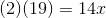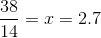## Example Questions

### Example Question #5 : How To Find If Two Acute / Obtuse Triangles Are Similar

The ratio of the side lengths of a triangle is 7:10:11. In a similar triangle, the middle side is 9 inches long. What is the length of the longest side of the second triangle?

12.1

9

7.7

9.9

10

9.9

Explanation:

Side lengths of similar triangles can be expressed in proportions. Establish a proportion comparing the middle and long sides of your triangles.

10/11 = 9/x

Cross multiply and solve for x.

10x = 99

x = 9.9

### Example Question #185 : Plane Geometry

Two triangles are similar to each other. The bigger one has side lengths of 12, 3, and 14.

The smaller triangle's shortest side is 1 unit in length.  What is the length of the smaller triangle's longest side?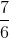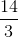Explanation:

Because the triangles are similar, a ratio can be set up between the triangles' longest sides and shortest sides as such: 14/3 = x/1.  Solving for x, we obtain that the shortest side of the triangle is 14/3 units long.

### Example Question #186 : Plane Geometry

The ratio of the side lengths of a triangle is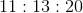. The longest side of a similar triangle is. What is the length of the smallest side of that similar triangle?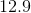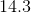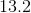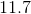Explanation:

Use proportions to solve for the missing side: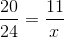Cross multiply and solve: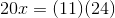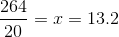### Example Question #187 : Plane Geometry

There are two similar triangles. One has side lengths of 14, 17, and 19. The smaller triangle's smallest side length is 2. What is the length of its longest side?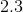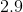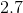Explanation:

Use proportions to solve for the missing side: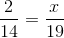Cross multiply and solve: